Miao, Y., Zhong, M., Liu, Z., and Sun, F. (2015). "Analysis of wood vibration energy attenuation based on FFT vibration signal," BioRes. 10(1), 272-281.

#### Abstract

The internal friction energy loss of vibration is an important indicator showing the vibrational performance of wood. This paper analyzed vibration signals based on the fast Fourier transform spectrum. The logarithmic average, logarithmic regression slope, and exponential function fitting methods were used to calculate the attenuation coefficient of friction energy of wood vibration in the full time and different time periods. The correlations of δ gained from different methods were compared and analyzed. The results showed that the linear correlations between different methods were significant in the entire period. The values obtained using the logarithmic average and logarithmic regression slope methods were similar. For different time periods, the rate of amplitude decay decreased over time. The values obtained using the logarithmic average method had the smallest fluctuation. In different time periods, the logarithmic average and logarithmic regression slope methods showed a significant linear correlation. However, the exponential function fitting method showed a low correlation with the logarithmic average and logarithmic regression slope methods.

Analysis of Wood Vibration Energy Attenuation Based on FFT Vibration Signal

Yuanyuan Miao, Minliang Zhong, Zhenbo Liu,* and Fengliang Sun

The internal friction energy loss of vibration is an important indicator showing the vibrational performance of wood. This paper analyzed vibration signals based on the fast Fourier transform spectrum. The logarithmic average, logarithmic regression slope, and exponential function fitting methods were used to calculate the attenuation coefficient of friction energy of wood vibration in the full time and different time periods. The correlations of δ gained from different methods were compared and analyzed. The results showed that the linear correlations between different methods were significant in the entire period. The values obtained using the logarithmic average and logarithmic regression slope methods were similar. For different time periods, the rate of amplitude decay decreased over time. The values obtained using the logarithmic average method had the smallest fluctuation. In different time periods, the logarithmic average and logarithmic regression slope methods showed a significant linear correlation. However, the exponential function fitting method showed a low correlation with the logarithmic average and logarithmic regression slope methods.

Keywords: Vibration performance; Wood; Internal friction energy loss; Attenuation coefficient

Contact information: Northeast Forestry University, Key Laboratory of Bio-based Material Science and Technology of National Ministry of Education, 26 Hexing Rd, Harbin, Heilongjiang, P.R. China 150040 China; *Corresponding author: liu.zhenbo@foxmail.com

INTRODUCTION

The vibration attenuation coefficient of wood, δ, is an important parameter of wood vibrational energy attenuation. This coefficient not only represents the degree of wood vibration energy attenuation but also has a crucial influence on wood vibration performance (Norimoto 1982; Ono and Norimoto 1983; Norimoto et al. 1986; Li 1995; Ilic 2001; Liu et al. 2005; Cui and Zhang 2006; Cui and Zhang 2009; Traore et al. 2010). By studying the attenuation coefficient, some vibration parameters would be obtained, such as vibration damping. Thereby, one can use nondestructive testing (NDT) to test wood defects. Chinese scholars have made use of the attenuation coefficient to calculate the wood vibration damping ratio. The number of knots in wood defects were determined by analyzing the damping ratio (Shen 2001). By utilizing formula between the attenuation coefficient and modulus of elasticity, one can calculate the modulus of elasticity. Thereby, it is possible to evaluate and classify the mechanical properties of wood (Kubojima et al. 1996, 1997; Shen and Liu 2001).

Based on previous research (Matsushita et al. 1984; Voichita 2005), the vibration attenuation coefficient of wood is an important parameter that has some influence on the accuracy of determination of other parameters in wood, such as damping ratio and modulus of elasticity. At present, some scholars have analyzed the attenuation coefficient in the entire time period only, which may be regarded as lacking in detail (Ono et al. 1983; Norimoto et al. 1986; Bakary et al. 2010; Kazuya et al. 2010). Vibration energy attenuation has two cases. One case represents linear processes of attenuation, whereas the other case is for nonlinear attenuation. As such, analyzing the attenuation coefficient over the entire time period only would enlarge the error of the resulting attenuation coefficient, which directly affects the accuracy of the damping ratio and modulus of elasticity. Piecewise analysis of vibrational energy could effectively reduce the error. It is of great significance for research of vibration performance and NDT to use the right method to analyze vibrational energy.

In this paper, a fast Fourier transform (FFT) analyzer was used to obtain the material vibration image. Excel calculations were used to analyze the time domain signal. Extracting the maximum and minimum values also means extracting the amplitude value. The logarithmic average, logarithmic regression of slope, and exponential function fitting methods were applied to compute the wood vibrational attenuation coefficient δ. Simultaneously, the attenuation coefficient δ was analyzed in different time periods. Finally, the difference and correlation of the attenuation coefficient δ obtained using different methods were compared and analyzed. By analyzing data from the entire time period and different time periods, one can derive the optimal calculation method of data of the attenuation coefficient δ in specific circumstances. This then allows analysis of the damping ratio and modulus of elasticity or other parameters based on most accurate attenuation coefficient δ.

EXPERIMENTAL

Materials

Good acoustic properties were observed for two tree species used in this experiment, namely, Picea jezoensis and Paulownia elongata S.Y. Hu. The samples were cut from the heartwood of 45-year-old Picea jezoensis and 20-year-old Paulownia elongata S.Y. Hu. They were dried to a moisture content of 12%, and conditioned at 20 °C and 65% relative humidity for 6 months before testing. A total of 66 specimens were used in this study. The sizes of the tree species are shown in Table 1.

Table 1. Various Parameters of the Specimens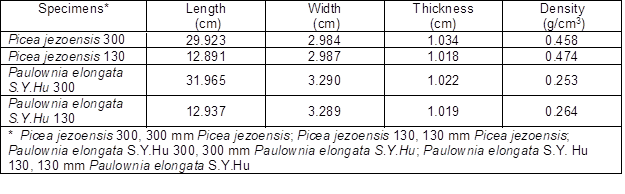Methods

The flexural vibration method was used in this work. Figure 1 shows that an elastic string was used for hanging the specimen horizontally on the wave-type node of clear lumber. Then, the node location was determined based on the theory of vibration. The vibrational mode of the fundamental wave (pseudo-primaries) was 0.224 times as long as the specimen from the node to the end. Initially, a small wooden hammer was used to knock on one end of the specimen or at its heart. A microphone was placed at the other end of the specimen to receive the signal. After this signal passed through the preamplifier and filter, the FFT analyzer was employed to process the time domain signal and frequency domain signal during vibration of the specimen.

Wood could vibrate under an outer impact force or a periodic force. After the external force disappeared, the damping vibration state commenced. The amplitude increased with time, as attenuation follows the proximately negative exponential law. When wood vibrates, vibrational energy would gradually decay because of internal friction. Attenuation speed is an important indicator showing wood acoustic vibration performance. In this research, the logarithmic average, logarithmic regression of slope, and exponential function fitting methods were applied to compute the wood vibrational energy attenuation coefficient.

To compute the internal friction index of wood vibrational energy, obtaining the amplitude value is critical. For each cycle of the original time domain data, the amplitude increases with time, as attenuation follows the proximately negative exponential law incompletely. As such, the attenuation coefficient of the vibration signal initially computed was inaccurate. Therefore, datapreprocessing was required. The moving average method was used for data preprocessing of the amplitude value, with the following calculation formula,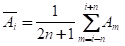（1）

where n is the number of moving points.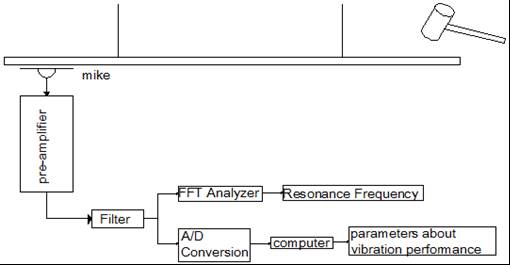Fig. 1. Wood vibration characteristic parameter test device

Logarithmic average method of the attenuation coefficient

(1) The logarithmic ratio of the specific value of the adjacent amplitude value after datapreprocessing was computed as follows: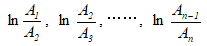(2)

(2) The amplitude value would be the average value of all logarithmic ratios that were obtained: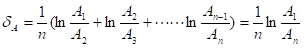(3)

Logarithmic regression of slope method of the attenuation coefficient

(1) The logarithmic of each amplitude value ratio after data preprocessing was computed as follows: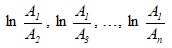(4)

(2) The quantities {1, 2, … , n-1} were set as the independent variable and the quantities{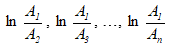}were set as the dependent variable. As such, the slope of the regression equations is the attenuation coefficient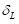of the logarithmic value.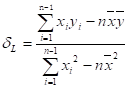(5)

x={1, 2, …, n-1}; y={} (6)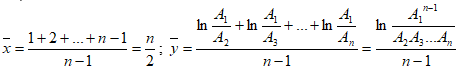(7)

Exponential function fitting method of the attenuation coefficient

Again the quantities {1, 2, …, n} served as the independent variable. Then, the preprocessed amplitude value {A1A2, …, An}were set as the dependent variables. These variables were computed on the basis of the exponential function, as follows: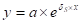(8)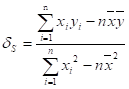(9)

x={1, 2, …, n}; y={ln A1, ln A2,…, ln An}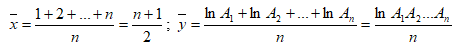(10)

Calculated in sections method of the attenuation coefficient

The vibrational time domain signal was divided into two or three phases based on time. Then, the corresponding attenuation coefficient was computed. A period of 0.04 s was devoted to data acquisition in the test for the 130 mm specimens. Time was divided into three phases, namely, 0.00 s to 0.01 s, 0.01 s to 0.02 s, and 0.02 s to 0.03 s. A period of 0.08 s was spent on data acquisition in the test for the 300 mm specimens. Time was divided into two phases, namely, 0.00 s to 0.04 s and 0.04 s to 0.08 s. The logarithmic average, logarithmic regression of slope, and exponential function fitting methods were applied to compute the wood vibrational attenuation coefficient in different time periods.

RESULTS AND DISCUSSION

Average Value of the Attenuation Coefficient by Different Computational Methods

Table 2 shows that the attenuation coefficient of Paulownia elongata S. Y. Hu was greater than that of P. jezoensis, and the attenuation coefficient of 130 mm specimens was greater than that of 300 mm specimens. Tables 3 and 4 show the average value of the attenuation coefficient in different time periods. The tables show that the rate of amplitude decay of different specimens decreased over time. The values obtained using the logarithmic average method had the smallest fluctuation.

Table 2. Average Value of the Attenuation Coefficient by Different Computational Methods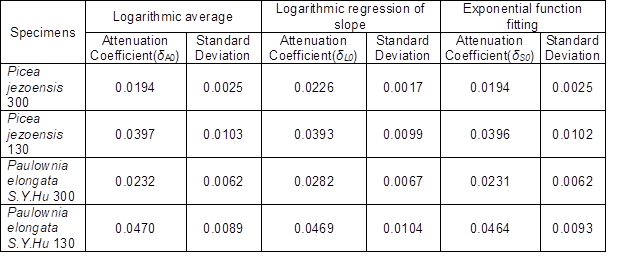Table 3. Average Value of the Attenuation Coefficient in Different Time Periods (130 mm Specimens)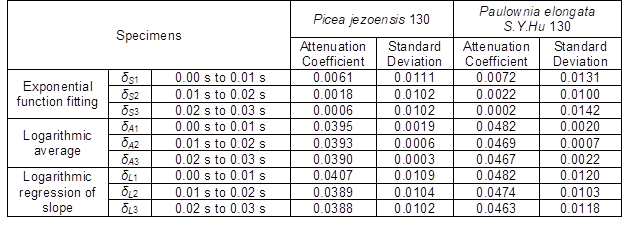Table 4. Average Value of the Attenuation Coefficient in Different Time Periods (300 mm Specimens)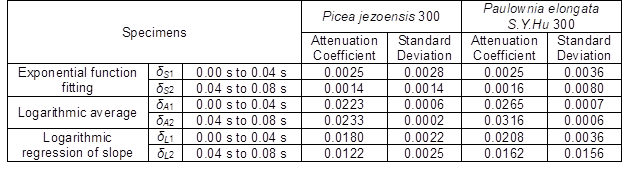Correlational Analysis of the Attenuation Coefficient by Different Computational Methods

Correlation analysis of the attenuation coefficient in the entire period

The logarithmic average, logarithmic regression of slope, and exponential function fitting methods were used to calculate the wood vibrational energy attenuation coefficient for 33 P. jezoensis specimens and 33 P. elongata S.Y.Hu specimens. The quantities δSδA, and δL were regarded as the corresponding attenuation coefficients. The one-variable linear regression equation was used to solve the question of the correlation analysis of the attenuation coefficient.

Figures 2 and 3 show that δSδA, and δL represented the linear correlations in these specimens. These attenuation coefficient values showed significant correlation, with values greater than 0.9. The correlation coefficient values of P. jezoensis were greater than those of P. elongata S.Y.Hu. For P. elongata S.Y.Hu, the correlation coefficients of δA and δL were highest (R2 = 0.9664), and the correlation coefficients of δS and δA were lowest (R2 = 0.9190). For P. jezoensis, the correlation coefficients of δS and δL were highest (R2 = 0.9997), and the correlation coefficients of δS and δA were lowest (R2 = 0.9741). Taking these factors into account and given that the correlation coefficients of δS and δL were closer to 1, the differences and correlations of the attenuation coefficients of δA and δL were compared and analyzed.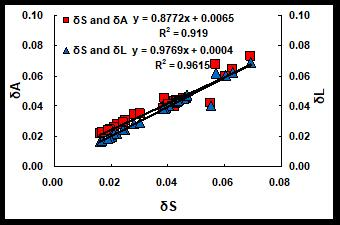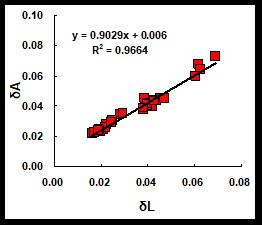(a) (b) Fig. 2. Correlation analysis of δA、δL and δS for Paulownia elongata S.Y.Hu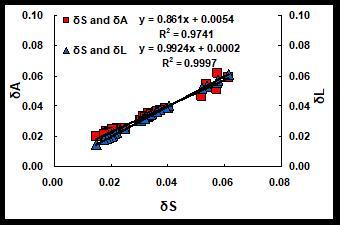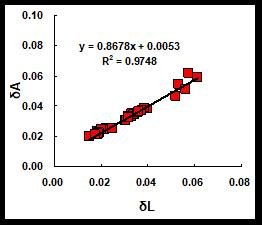(a) (b) Fig. 3. Correlation analysis of δA、δL and δS for P. jezoensis

Correlation analysis of δA and δL in different time periods

Using different bandwidths to determine vibration signal in the experiment, the 130 mmspecimens were different from the 300 mm specimens with regard to the vibration signals obtained during different time periods of data acquisition. For more scientific results, different attenuation coefficients were compared using different lengths of specimens. A time period of 0.04 s was employed for data acquisition in the test for the 130 mm specimens. The specimens were compared and analyzed values for three time periods. A period of 0.04 s was used for data acquisition in the test for the 300 mm specimens. Data were then compared and analyzed values for two time periods, namely, 0.00 s to 0.04 s and 0.04 s to 0.08 s.

Figures 4 to 7 show that δA and δL represented the linear correlations in these specimens in different time periods. The smallest correlation coefficients were obtained in the 0.02 s to 0.03 s period of the 130 mm P. elongata S.Y.Hu, 0.00 s to 0.04 s period of the 300 mm P. elongata S.Y.Hu, and 0.00 s to 0.04 s period of the 300 mm P. jezoensis. The other correlation coefficient values were greater than 0.9. In the 0.02 s to 0.03 s period, the correlation coefficient of the 130 mm P. elongata S.Y.Hu was lower than that of the 130 mm P. elongata S.Y.Hu.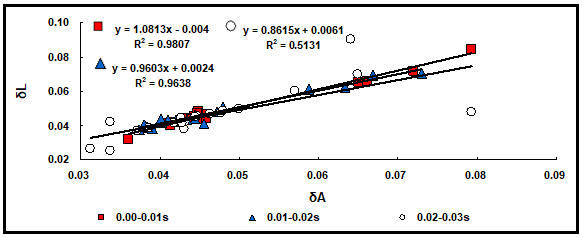Fig. 4. Correlation analysis of δA and δL for the 130 mm Paulownia elongata S.Y.Hu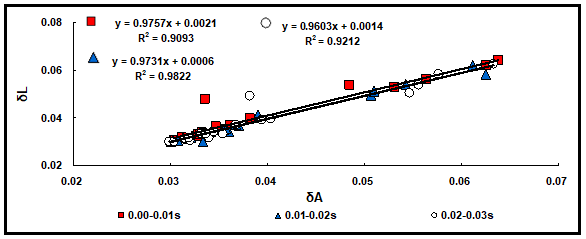Fig. 5. Correlation analysis of δA and δL for the 130 mm P. jezoensis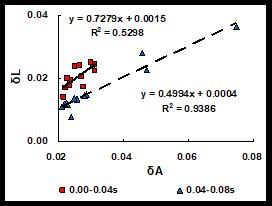Fig. 6. Correlation analysis of δA and δL for the 300 mm Paulownia elongata S.Y. Hu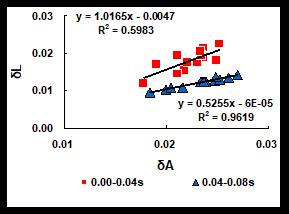Fig. 7. Correlation analysis of δA and δL for the 300 mm P. jezoensis

The other correlation coefficient values were similar in other time periods. Different tree species have different attenuation amplitudes. The 300 mm P. elongata S.Y. Hu and 300 mm P. jezoensishad low correlation coefficients (R2 = 0.52 to 0.60) for δA and δL. These results show that the attenuation image was significantly changed at the initial stage. As such, a suitable computationalmethod had to be selected.

CONCLUSIONS

The logarithmic average, logarithmic regression of slope, and exponential function fitting methods were used to calculate the wood vibrational energy attenuation coefficient of friction energy in the full time and different time periods. By analyzing these different attenuation coefficients, the following conclusions could be drawn:

1. Generally, considering the average value of the attenuation coefficient obtained using the three methods, δA was similar to δS, whereas δL was significantly different from the other two values. For the entire period, the logarithmic average method was selected as the most suitable way to compute the attenuation coefficient when the vibration image shows a linear correlation.
2. For the entire time period, values computed using the exponential function fitting, logarithmic average, and logarithmic regression of slope methods had significant correlation coefficient values close to 1. This result showed slight differences between these three methods.
3. By comparing and analyzing the correlation coefficient values, a significant change was observed in the vibration signal of time domain at the initial stage. For the nonlinearvibration signal, the logarithmic regression of slope or exponential function fitting method should be selected. For the linear vibration signal, the logarithmic average method is recommended.

ACKNOWLEDGMENTS

The authors are grateful for the support by the Fundamental Research Funds for the Central Universities (DL12EB03-01), and the special fund project of Harbin science and technology innovation talents research (RC2012LX-002001) for this research.

REFERENCES CITED

Cui, Y. Y., and Zhang, H. J. (2006). “Research on the relationship between the number of knots and wood modal parameters,” Beijing Institute of Mechanics, The 12th Annual Conference, 184-186.

Cui, Y. Y., and Zhang, H. J. (2009). “Lateral vibration measurement structural timber modulus of elasticity,” Practical Forestry Technology (12), 57-59.

Ilic J. (2001). “Relationship among the dynamic and static elastic properties of air dry Eucalyptus delegatensis R. Baker,” Holz als Roh-und Werkstoff 59(3), 169-175. DOI: 10.1007/s001070100198.

Kazuya, M., Yuji, K., Iris, B., Shiro, S., and Eiichi, O. (2010). “Extractives of muirapiranga (Brosimun sp.) and its effects on the vibrational properties of wood,” Journal of Wood Science56(1):41-46. DOI: 10.1007/s10086-009-1051-3.

Kubojima, Y., Yoshihara, H., Ohta, M., and Okano T. (1996). “Examination of the method of measuring the shear modulus of wood based on the Timoshenko’s theory of bending,” Mokuzai Gakkaishi 42(12), 1170-1176.

Kubojima, Y., Yoshihara, H., Ohta, M., and Okano T. (1997). “Accuracy of the shear modulus of wood obtained by Timoshenko’s theory of bending,” Mokuzai Gakkaishi 43(5), 439-443.

Li, J. (1995). Research of Chinese Timber, Northeast Forestry University Press, Harbin, China.

Liu, Z. B., Liu, Y. X., Yu, H. P., Yuan J.Q. (2005). “Research on the dynamic modulus of elasticity measurement of lumber,” Scientia Silvae Sinicae 41(6), 126-131.

Matsushita, K., Kuratani, S., Okamoto, T., and Shimada, M. (1984). “Young’s modulus and internal friction in alumina subjected to thermal shock,” Journal of Materials Science Letters3(4), 345-348.

Norimoto, M. (1982). “Structure and properties of wood used for musical instruments I. Selection of wood used for piano soundboards,” Mokuzai Gakkaishi 28(7), 407-417.

Norimoto, M., Tanaka, F., Ohgama, T. Ikimune R. (1986). “Specific dynamic Young’s modulus and internal friction of wood in the longitudinal direction,” Mokuzai Gakkaishi 22, 53-65.

Ono, T., and Norimoto, M. (1983). “Study on Young’s modulus and internal friction of wood in relation to the evaluation of wood for musical instruments,” Japanese Journal of Applied Physics22, 611-614.

Shen, J. (2001). “Study on relationships between Picea species structures and vibration properties,” Northeast Forestry University.

Shen, J., and Liu Y. X. (2001). “Advances in study and research on acoustic vibration property of wood,” World Forestry Research 14(1), 30-36.

Traore, B., Brancheriau, L., Perre, P., Stevanovic, T., and Diouf, P. (2010). “Acoustic quality of vène wood (Pterocarpus erinaceus Poir.) for xylophone instrument manufacture in Mali,” Annals of Forest Science 67(8), 815. DOI: 10.1051/forest/2010054.

Voichita, B. (2005). Acoustics of Wood, Springer-Verlag Berlin and Heidelberg GmbH & Co. K, Boca Raton, USA. 176-187. DOI: 10.1007/3-540-30594-7.

Article submitted: August 20, 2014; Peer review completed: October 15, 2014; Revised version received and accepted: November 10, 2014; Published: November 17, 2014.